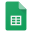# Final Assessment

Congratulations, you've reached the end of the module! To sum it up, you will demonstrate your LaTeX skills by typesetting a short document with a definition, a theorem, and a proof, all related to integral calculus.

## Instructions

Starting from a Blank Project on Overleaf, use LaTeX to typeset the definition, theorem, and proof shown in the PDF below. You need not add or change anything from the document - this activity is assessing your ability to typeset content in LaTeX, not your mathematical or writing skills.

Specific requirements for the document:

• All mathematical expressions should be reproduced accurately, in the style (inline or display) in which they appear in the document.

• Copy formatting (font changes, paragraph breaks, sections) from the sample.

• Put your own name as the author and today's date as the date.

Specific requirements for the code:

• Use the package amsmath.

• Place multiple lines of equations in an aligned environment.

As you work, you may refer back to the tutorial, or any resources of your choice. Have a look at the Command List and Resources page. Consider using Detexify if you encounter any unfamiliar symbols.

When you are done, ensure that your project is free of errors, then export your project as a PDF.

Submit both the PDF and your code as a .tex file in the form at the bottom of this page.

## Sample documentLaTeX_Final_Document.pdf

### Raw text for document

You may copy and paste the following text to save time typing while creating the final document. Some simpler expressions have been "converted" to plain text here, the more complex expressions are replaced with [see sample document for expression].

Definition of the Definite Integral

Let f(x) be a function defined for a <= x <= b. Divide the interval [a, b] into n subintervals of equal width, Delta x = (b - a)/n. Let xi* be a point from the ith subinterval.

Then, the definite integral of f from a to b is [see sample document for expression] provided that this limit exists.

The Fundamental Theorem of Calculus

Theorem

First Fundamental Theorem of Calculus. Suppose the function f is continuous on the interval [a, b]. Then the function g defined by [see sample document for expression] is differentiable on (a, b), and [see sample document for expression].

Proof

Using the limit definition of the derivative, we have

[see sample document for expression]

Applying the linear properties of the definite integral, we simplify the expression to

[see sample document for expression]

The Mean Value Theorem for Integrals states that if f is continuous over [a, b], then there is a point c with a <= c <= b such that [see sample document for expression].

Applying the Mean Value Theorem for Integrals on the interval [x, x+h], there must be a point c with x <= c <= x + h such that [see sample document for expression]. Furthermore, as h -> 0, we have c -> x. Thus,

[see sample document for expression].

## Submission formFinal Document Rubric